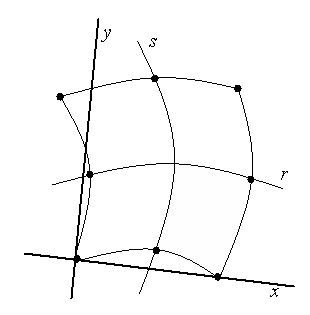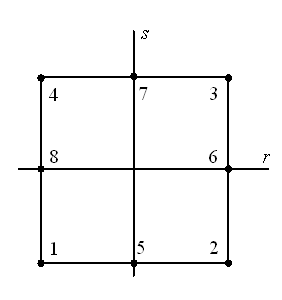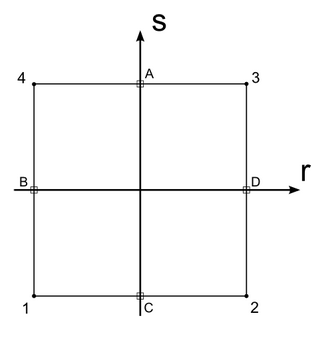# # Element Stiffness

To generate a stiffness matrix for a curvilinear quadrilateral or triangular element a new approach must be used. Most finite element codes used an approach based on isoparametric or similar elements.

In an isoparametric element the element displacements are interpolated in the same way as the geometry, e.g. a plane stress element. In a superparametric or degenerate isoparametric element the interpolation on the geometry is of a higher order than the interpolation of the displacements, e.g. a plate element. In a subparametric element the interpolation of the geometry is of a lower order than the interpolation of the displacements, e.g. an eight noded straight sided quadrilateral element, where a different geometric interpolation function is used for the geometry from that for the displacements. The term isoparametric is often used as a general term to cover all these element types.

For a plane stress problem, we can establish a material matrix that relates stress and strain. The displacements in a local coordinate system are

the strains are

and the stresses are

For an elastic-isotropic material the material matrix is

where are the Young’s modulus and Poisson’s ratio respectively.

Note that there is an out of plane strain , which we can ignore as it plays no part in the element formulation.

The strains are defined in terms of the displacements as

The simplest elements to consider are the 4 noded and 8 noded quadrilateral elements, of which the 4 noded element can be considered a simplification of the 8 noded element. A typical 8 noded element is shown below. The element has an arbitrary local coordinate system based on the location of the nodes and the element property axis , and a natural (curvilinear) coordinate system based on the topology of the nodes.We can set up interpolation functions to interpolate the geometry as follows

where the are the interpolation functions defined below.

These interpolation functions are chosen so that

As the elements are isoparametric we use the same interpolation function for the displacements so the displacement in the element is related to the nodal displacements by

To evaluate the stiffness matrix we need the strain-displacement transformation matrix. The element displacements are obtained in terms of derivatives of the element displacements with respect to the local coordinate system . Because the elements displacements are in the natural coordinate system we need to relate the derivatives in the local coordinate system to those in the natural coordinate system. We can write an equation for the derivative with respect to in terms of derivatives with respect to

to establish these derivatives we use the chain rule to set up the following relationship

or in matrix notation

where is the Jacobian operator relating natural coordinate derivatives to local coordinate derivatives. Given that we know and in terms of the interpolation functions the Jacobian operator is easily found

This requires that the inverse of the Jacobian exist, which requires that there is a one to one correspondence between natural and local coordinates. This will be the case provided the element is not grossly distorted from a square and that it does not fold back on itself.

We can then evaluate

and thus we can construct the strain-displacement transformation matrix,

where is the vector of nodal displacements. The element stiffness corresponding to the local element degrees of freedom is then

The elements of are functions of the natural coordinate system, . Therefore the volume integration extends over the natural coordinate volume so the volume differential needs to be written in terms of the natural coordinates

The volume integral is not normally amenable to an explicit integration so normally a numerical integration technique is used. The integral can be written

where

and the integral is performed in the natural coordinate system of the element. This is convenient as the limits of the integration are then ±1. The stiffness can then be calculated

where the matrix is evaluated at the Gaussian integration points and are Gaussian weights.

In a similar way the mass matrix and the load vectors are established.

where is a matrix of interpolation functions and , are the body force vector, surface force vector and initial stress vector respectively.

## # Geometric stiffness matrix of shell element

The geometric stiffness matrix is calculated from:

where

With are the in-plane forces of the shell element in , and shear directions respectively.

and is the number of nodes of the elements

## # 2D Plate Elements

The formulation of 2D plate and shell elements considers both in-plane and transverse (out-of-plane) displacements. Following Mindlin-Reissner plate theory, in addition to the bending strains we consider the effect of transverse shear strain in our complete expression for the element strain

where is the out-of-plane displacement and is introduced as an independent variable to express the section rotations (i.e. rotations of the transverse normals about the local and axes).

We can define separate material matrices and that relate stress and strain for the pure bending and shear strains respectively and so the pure bending moments and shear forces can be written

and

respectively.

In this way we can then express the local element stiffness matrix as a summation of the in-plane and out-of-plane stiffness’s

where and represent the strain-displacement transforms for the bending and shear components respectively.

While brief, this outlines the basic approach to the Mindlin-Reissner 2D element stiffness formulation. In GSA we label this concisely as the Mindlin formulation.

## # MITC Element Formulation

We find that the Mindlin formulation is an effective approach for 2D parabolic elements where the 8-noded element accommodates sufficient terms in the stiffness matrix to sufficiently define the behaviour of the element numerically. However the same formulation defined over a linear element becomes noticeably more problematic where the absence of available terms in our 4-noded stiffness matrix leads to numerical difficulties in expressing the same element behaviour. Specifically we find a difficulty in attempting to represent the transverse shear strain terms. Analytically as before we can express the transverse shear strain as

although numerically, the difference in the order of terms for the shear strain may lead to artificial stiffening of the element where the shear terms are numerically constrained from approaching zero. See reference 1 in the bibliography for further information. This restriction would be particularly noticeable where the thickness of the plate is small.

A widely practiced remedy is to under-integrate the shear term and while effective, its use is at the cost of reduced accuracy and stability for the element. The problem of stability alone is often of greatest concern where the phenomenon of hourglassing can become apparent in elements where the thickness to length ratio is large.

An alternative formulation was put forward by Bathe and Dvorkin and has been found to be especially effective at resolving these difficulties. The formulation is extendable to higher order elements although we find the approach is most effective when resolving the difficulties most apparent in linear elements. The formulation is based upon the theory of Mixed Interpolated Tensoral Components (MITC). For the pure displacement-based Mindlin formulation we use the independent variables

where Bathe now introduces a separate independent variable to represent the transverse shear term

We use to represent an additional set of interpolation functions for our new variable which we find by a direct evaluation of the shear strain at points , that is

For a linear 2D element we obtain direct values for the shear strain at four points A, B, C, D on the element and so we evaluate the displacement and section rotations at these points through direct interpolation.We can then construct the interpolations

using direct values for the shear strains obtained at the four points. This replaces our original expression for the shear terms and we continue to construct the local stiffness matrix as normal in a similar approach as before in 2D isoparametric elements. It is lastly worthwhile to note that the interpolation above assumes our element is in the isoparametric coordinate system. Further transformations are necessary to interpolate the shear strains directly through an arbitrary element in local space. Indeed, when this is done the element shows considerably improved predicative capabilities for distorted elements.

## # Element shape functions

The element shape functions for 2D elements are

These are for the different element shapes

Tri-3

Tri-6

## # 2D Element Shape Checks

A number of element checks are carried out by GSA prior to a GSS analysis. Other analysis programs may have different limits but the same principles apply. For GSS the following warnings, severe warnings and errors are produced.

### # Triangle

Warning Severe warning Error

Warning Severe warning Error

where

longest side / shortest side

minimum angle

maximum angle

distance out of the plane of the element of edge 1 / longest side

Notes:

The distance out of plane of edge 1 is calculated as

Where is the element normal, , are the coordinates of the first and second corner nodes and is the length of the longest side of the element.

Mid-side node locations not checked but should be approximately halfway along edge.

No check on ratio thickness/shortest side.

## # Hourglassing

When Quad 4 elements with the Mindlin formulation are used in bending it is possible to encounter hourglassing problems. This is a problem that arises with under-integrated elements where there are insufficient stiffness terms to fully represent the stiffness of the element. The problem is noticeable in the results by an hourglass pattern in the mesh as shown below.This problem is avoided using the MITC formulation for Quad 4 elements. This formulation uses a separate interpolation method for the transverse shear strains and provides considerably greater stability than the original Mindlin method. The original Mindlin method is kept in GSA for compatibility with previous models although for new models the MITC formulation is recommended.

Alternatively when parabolic accuracy is required Quad 8 elements are recommended. These also formulate elements that are still stiff in all modes of deformation, even when under-integrated.

## # 3D Element

The element shape functions for 2D elements are

These are for the different element shapes

Tetra-4

Pyramid-5

Wedge-6

Brick-8